Examples

Chapter 15 Class 9 Probability (Deleted)
Serial order wise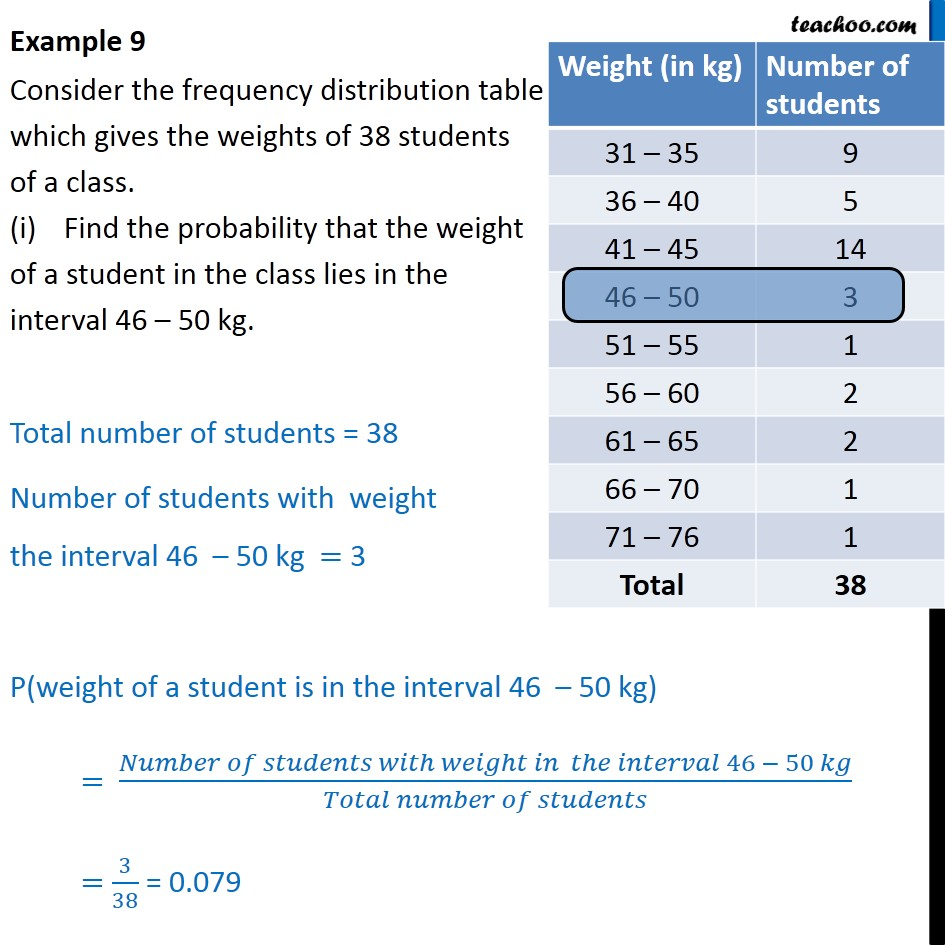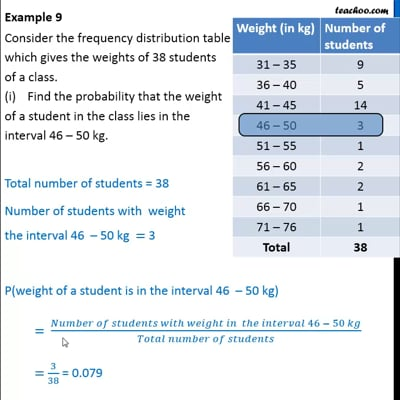This video is only available for Teachoo black users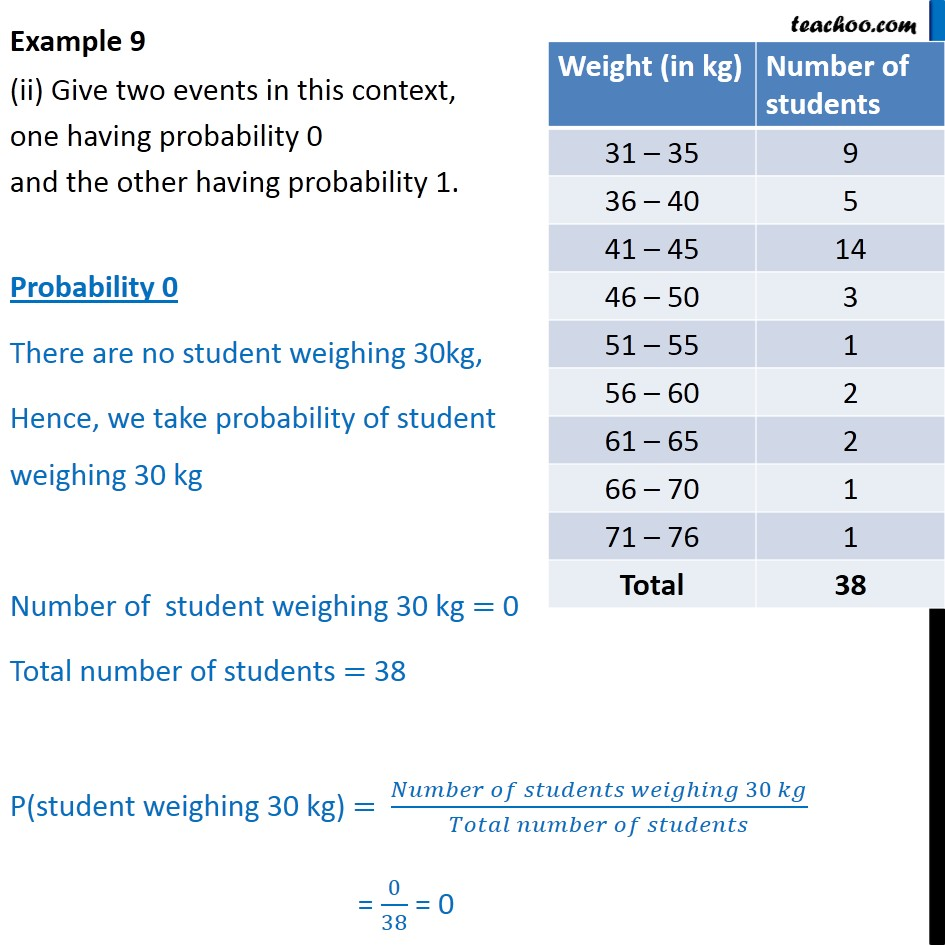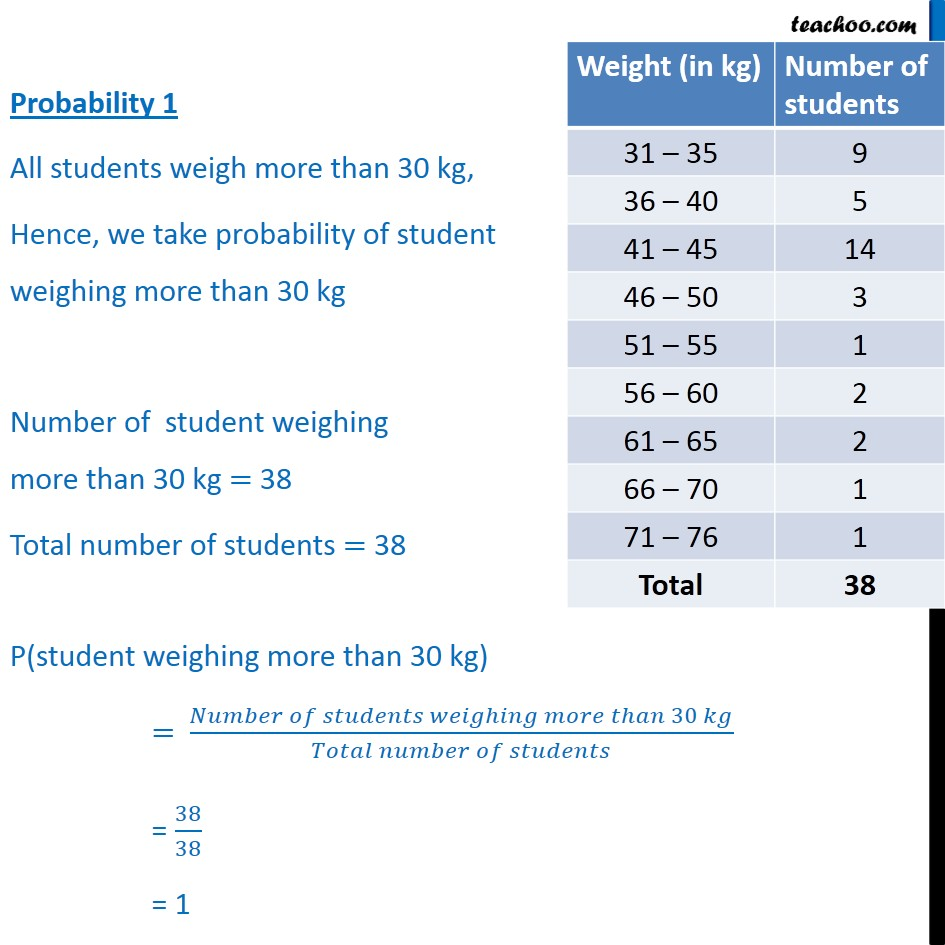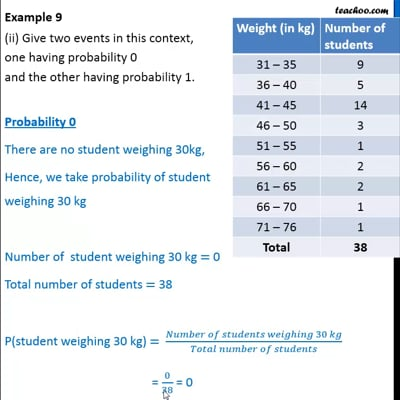This video is only available for Teachoo black users

Maths Crash Course - Live lectures + all videos + Real time Doubt solving!

### Transcript

Example 9 Consider the frequency distribution table which gives the weights of 38 students of a class. Find the probability that the weight of a student in the class lies in the interval 46 – 50 kg. Total number of students = 38 Number of students with weight in the interval 46 – 50 kg = 3 P(weight of a student is in the interval 46 – 50 kg) = (𝑁𝑢𝑚𝑏𝑒𝑟 𝑜𝑓 𝑠𝑡𝑢𝑑𝑒𝑛𝑡𝑠 𝑤𝑖𝑡ℎ 𝑤𝑒𝑖𝑔ℎ𝑡 𝑖𝑛 𝑡ℎ𝑒 𝑖𝑛𝑡𝑒𝑟𝑣𝑎𝑙 46 − 50 𝑘𝑔)/(𝑇𝑜𝑡𝑎𝑙 𝑛𝑢𝑚𝑏𝑒𝑟 𝑜𝑓 𝑠𝑡𝑢𝑑𝑒𝑛𝑡𝑠) = 3/38 = 0.079 Example 9 (ii) Give two events in this context, one having probability 0 and the other having probability 1. Probability 0 There are no student weighing 30kg, Hence, we take probability of student weighing 30 kg Number of student weighing 30 kg = 0 Total number of students = 38 P(student weighing 30 kg) = (𝑁𝑢𝑚𝑏𝑒𝑟 𝑜𝑓 𝑠𝑡𝑢𝑑𝑒𝑛𝑡𝑠 𝑤𝑒𝑖𝑔ℎ𝑖𝑛𝑔 30 𝑘𝑔)/(𝑇𝑜𝑡𝑎𝑙 𝑛𝑢𝑚𝑏𝑒𝑟 𝑜𝑓 𝑠𝑡𝑢𝑑𝑒𝑛𝑡𝑠) = 0/38 = 0 Probability 1 All students weigh more than 30 kg, Hence, we take probability of student weighing more than 30 kg Number of student weighing more than 30 kg = 38 Total number of students = 38 P(student weighing more than 30 kg) = (𝑁𝑢𝑚𝑏𝑒𝑟 𝑜𝑓 𝑠𝑡𝑢𝑑𝑒𝑛𝑡𝑠 𝑤𝑒𝑖𝑔ℎ𝑖𝑛𝑔 𝑚𝑜𝑟𝑒 𝑡ℎ𝑎𝑛 30 𝑘𝑔)/(𝑇𝑜𝑡𝑎𝑙 𝑛𝑢𝑚𝑏𝑒𝑟 𝑜𝑓 𝑠𝑡𝑢𝑑𝑒𝑛𝑡𝑠) = 38/38 = 1